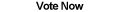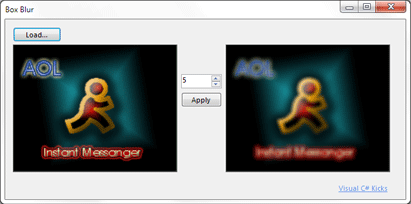Home Articles Components Code Snippets Links# C# Box Blur## Box Blur

In this article we'll take a look at how to implement an image box blurring effect in C#.

Box blurring is accomplished with image convolution, a concept that applies to a wide range of image filters.

## Convolution

A convolution is a linear operation on a signal and a kernel. In this case the signal is the image and the kernel is the filter. More specifically, in discrete image processing, convolution is the dot product of every point in the signal with the kernel. So a very simple example:

```signal: [1, 2, 3, 4]
kernel: [5, 6, 7]
convolution: [1 * 5 + 2 * 6 + 3 * 7,
2 * 5 + 3 * 6 + 4 * 7,
3 * 5 + 4 * 6 + 0 * 7,
4 * 5 + 0 * 6 + 0 * 7]  ```

This is a very simple example but it shows the basics of convolution. Notice that it takes a considerable amount of operations, in this case our simple 4x1 convolved with 3x1 took 12 multiplications and 8 additions. This will be important to consider. Also notice that near the edge we run out of samples in the signal to do the convolution properly, so the calculations are done with 0's. Zero-padding is only one of many possible ways to address the issue.

## Box Kernel

To blur an image we need to apply a 2D convolution (since images have width and height). The question is what the kernel will look like. With the right kernel, we can simply convolve it with any image to blur it.

To come up with the kernel, we need some simple insight into the convolution process. As described above, every pixel is the dot product of the kernel and the area around that pixel. In other words, the kernel will dictate how a single pixel is combined with the pixels around it. So here is an example of a kernel:

```[0, 0, 0]
[0, 1, 0]
[0, 0, 0]```

Assume the kernel is centered on the each pixel when it's applied to the image. What will happen? Absolutely nothing. This kernel results in a value that consists entirely of the current pixel (value 1) and nothing of neighboring pixels (value 0). (This kernel is called a delta by the way).

Now let's take a look at another kernel:

```[0,  0,   0 ]
[0, 0.5, 0.5]
[0,  0,   0 ]```

Take a second to think about it. In this case, the resulting pixel is a combination of the half of the value of the current pixel and half of the value of its right neighbor. This is a blur because each pixel is spread out with its neighbor.

If we apply a similar kernel to every single pixel, we are doing something very similar to averaging the values of pixels within a neighborhood. Averaging reduces the sharpness of the overall image, thus we get a blur.

So we finally get a box blur kernel:

```[1/9, 1/9, 1/9]
[1/9, 1/9, 1/9]
[1/9, 1/9, 1/9]```

This kernel will evenly spread out the values of each pixel in an image. How do we control the amount of blurring? The size of the kernel. A bigger kernel will average a larger area, thus reducing sharpness more. A small kernel will blur much less.

## Final Notes

The sample application uses the FastBitmap class, which encapsulates the logic for faster image processing in C#. You will also notice that there are two blurring functions, BoxBlur and FastBoxBlur. The difference is BoxBlur applies the single box blur kernel described above. FastBoxBlur uses a property of convolution called filter separability which quickly explained means that we can split the single box kernel into two smaller ones. Then two convolutions are applied in succession with those smaller filters. The result is the same but the total number of operations is much much less.

Another thing to know is that a box blur is only one of many blurs. A popular blur is called a Gaussian blur, which works exactly the same way (including the filter separability to make it fast). The only difference is that each pixel is not evenly spread out with all its neighbors, instead pixels closer to the center have a greater weight.

Back to C# Article List

Other C# Articles